# Nash theorems (in differential geometry)

(diff) ← Older revision | Latest revision (diff) | Newer revision → (diff)
1) Nash' theorem on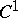-imbeddings and-immersions. An immersion (imbedding)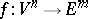of classof an-dimensional Riemannian spacewith metricof classinto an-dimensional Euclidean space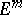is called short if the metric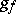induced by it on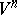is such that the quadratic form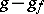is positive definite. Ifhas a short immersion (imbedding) in,, thenalso has an isometric immersion (imbedding) of classin. Under the restriction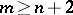this theorem was proved in , and in the form stated above in . This theorem implies, in particular, that if a compact Riemannian manifoldhas a-imbedding (immersion) in,, thenalso has an isometric-imbedding (immersion) in. Another consequence of Nash' theorem is that every point ofhas a sufficiently small neighbourhood that admits an isometric imbedding of classin.
2) Nash' theorem on regular imbeddings. Every compact Riemannian manifoldof class,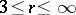, has an isometric-imbedding in, where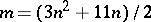. Ifis not compact, then it has an isometric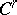-imbedding in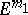, where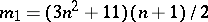.
Nash' theorem on regular imbeddings results from an application of Nash' implicit-function theorem on the inversion of a broad class of differential operators. The meaning of this theorem is that when a certain linear algebraic system of equations connected naturally with a differential operatoris solvable and when a reasonable topology is introduced in the image and inverse image, then the operator in question is an open mapping, that is,is locally invertible near any point of its range. For the equations of an imbedding of a Riemannian manifold in a Euclidean space this reduces to the fact that the first and second derivatives of the mappingwith respect to the intrinsic coordinates ofmust be linearly independent. Such imbeddings were first considered in ; they are called free. Nash' implicit-function theorem implies that a compact Riemannian manifoldsufficiently close to another one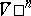having a free imbedding inalso has a free imbedding in. This fact and the original method of extension with respect to a parameter lead to Nash' theorem on regular imbeddings (see ). By extending Nash' method to non-compact manifolds and analytic imbeddings, and also by a principal refinement of the process of extension with respect to a parameter, it has been proved that every infinitely-differentiable (analytic) Riemannian manifoldhas an isometric differentiable (analytic) imbedding in, where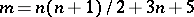(see ).